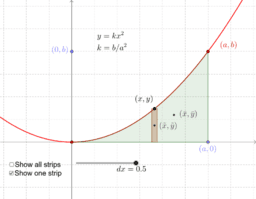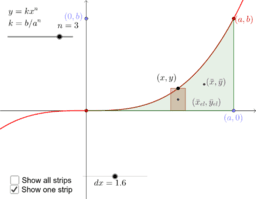## Determination of Centroids by Integration

### Handout SolutionsParabolic Spandrel: $y=kx^2$General Spandrel: $y=kx^n$
In this problem the width and height are given explicitly, where I would use $a$ and $b$, and she uses $\tilde{x}$ for what I call $\bar{x}_{el}$ and similarly for $\tilde{y}$.